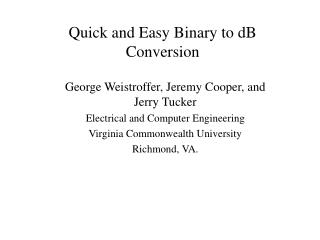Download PresentationQuick and Easy Binary to dB Conversion

# Quick and Easy Binary to dB Conversion - PowerPoint PPT PresentationDownload Presentation## Quick and Easy Binary to dB Conversion

- - - - - - - - - - - - - - - - - - - - - - - - - - - E N D - - - - - - - - - - - - - - - - - - - - - - - - - - -
##### Presentation Transcript

1. Quick and Easy Binary to dB Conversion George Weistroffer, Jeremy Cooper, and Jerry Tucker Electrical and Computer Engineering Virginia Commonwealth University Richmond, VA.

2. New algorithm for converting binary integers to decibels (dB) • Advantages over known alternative methods • Faster • No real-time floating point operations • Only real-time arithmetic is one integer subtraction • Requires fewer resources (i.e. less memory) • Simple to implement • Limitations • Reduced precision • High precision is not typically needed for dB’s • Precision can be increased at the cost of more memory.

3. Motivation Binary output samples of analog input voltages. Level detector V1 Analog Mux n-bit ADC w, n-bit word Level detector V2  Level detector dB Vk V1,V2,…Vk

4. The problem Consider an unsigned binary integer w expressed as • Typically w is the output of an n-bit ADC and is changing in real-time. Let G be the comparison in dB of w to the maximum value of w. Then G Changes in real time Fixed – depends only on number of bits in w.

5. For any particular value of n, the Gc term is a constant and does not have to be computed in real time.

6. Computing 20log10(w) • The difficulty is the evaluation of the 20log10(w) term. • The input w changes for each sample. • 20log10(w) must continually be recalculated. • A fast method is needed for this calculation. • Such a method can be developed by taking advantage of the fact that dB values typically do not need to be represented with high precision. • Therefore, it is possible to use an approximation for w.

7. The approximation of w • Then assuming w≠ 0, w can be expressed as where bit m with m < n is the left-most (most significant) “1” of w. • To approximate w, use only the first r-bits to the right of the most significant “1” of w. • Let wmin = (1wm-1wm-2…wm-r0…0)2 and wmax = (1wm-1wm-2…wm-r 1…1)2 • Then wmin≤ w ≤ wmax Note that when m ≤ r then wmin = w =wmax. • w wmin= (1wm-1wm-2…wm-r0…0)2 Bit m r-bits

8. The approximation of G Recall that G = 20log10(w)-20log10(2n-1) Therefore, Gmin = 20log10(wmin)-20log10(2n-1) Using G  Gmin and letting R = (wm-1wm-2…wm-r)2 it is easy to show that G can be approximated using three terms. where Depends only on m Depends only on R A constant

9. The approximation error The worst case error occurs when m=n-1. Using The error will never exceed this worst case error.

10. Refinements to the algorithm • Combine the Gc value with each term in the Gm table. • This is slightly more efficient than subtracting the Gc value each time a dB value is computed. • Scale table values to avoid fractional values. • Let k be the scale factor. • If resolution is ½ dB let k = 2, if resolution is ¼ dB let k = 4, etc. • Represent the negation of G as an unsigned integer. • G is always negative. Therefore, evaluate –kG  k(GC – Gm) - kGR

11. Example: Let w = (1 0101 01011000011)2 m = 15, R = (0101)2 = 5, 2(Gc – Gm(15)) = 12, 2GR(5) = 5 -2G  12 – 5 = 7, G  -3.5 dB, Actual value G = -3.517 dB

12. Conclusion • A technique that is fast, easy to implement, and memory efficient has been developed for converting a binary integer to dB’s. • It takes advantage of the fact that in practice decibels do not need to be calculate to high precision. • It uses two small lookup tables to find the dB values. • The method has been illustrated for the specific case where w is a 16-bit unsigned integer, and where four bits following the most significant “1” of w are used to approximate w. • Requires two 16 byte tables. • The dB value obtained is always within ½ dB of the actual value. • The algorithm has been implemented and verified using both 8051 assembly language and VHDL.